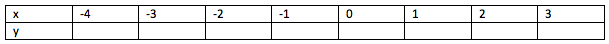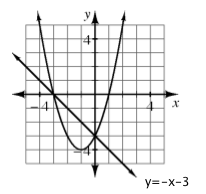### Home > GC > Chapter 2 > Lesson 2.2.4 > Problem2-92

2-92.

Graph the following equations on the same set of axes. Label each line or curve with its equation. Where do the two curves intersect? 2-92 HW eTool (Desmos).

$y = -x -3$        $y = x^2 + 2x-3$

Use the eTool below to graph the equations and view the intersection.
Click the link at right for the full version of the eTool: GC 2-92 HW eTool

Make a table to help you graph $y = x² + 2x - 3$.They intersect at ($-3, 0$) and ($0, -3$).